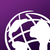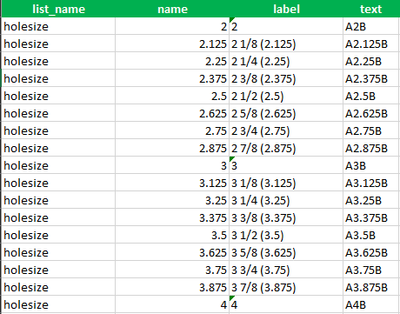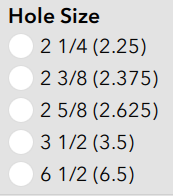# Dynamic Choice_Filter Created From Repeat Select_One Question

819
6
01-05-2021 12:52 PMNew Contributor III

Hello

I have searched high and low for months regarding what I am attempting to accomplish.  I have finally decided to post a question first, and if it is not possible, will post it as an idea.  I'll do my best to describe what I am trying to do.

I have a repeat select_one question that pulls from a list in the choices tab.  In a separate select_one question outside of the repeat, which pulls from the same choice list, I want it to be filtered based on the values from the repeat select_one question.  For example, if the choice list values are 1 thru 10, and only the values of 4, 5, and 6 were selected in the repeat question, the separate select_one question choice list would only have 4, 5, and 6 from which to choose.

Is this possible?  Any ideas on how one would accomplish this?  Thank you.

2 Solutions

Accepted SolutionsbyMVP Notable Contributor

First I would wonder why you need a pick list if you already know the 3 values, just fill them in as text.  But I am guessing there is a reason.

I had a coworker come up with a funky way.  For us the lists were 6,000+ so this got to slow, but it did work.

Add a calculate field that does a join of all the choices from your repeat.

Field name = SpeciesListJoin  calculation is  join(",",\${AddPlants})

Then use this list in the choice filter of your other question

Field type select_one_from_file itemsets.csv  choice filter regex(\${SpeciesListJoin}, name)

Hope that helps - I am still not really sure how it works but it seems to.New Contributor III

@DougBrowning I figured it was the decimal causing issues, but after much testing determined it was both the decimal and numbers over one digit causing an issue.  So I kept at it and found a solution.  It might not be the best, but it works.

I created another column in the choices tab called "text", and concatenated an A to the front of the value in the name column, and a B at the end.

Then I added another field in the survey that concatenates the A and B to the select_one answer.

Next I changed the calculate field with the formula join(",", \${select_one}) to the field that does the concatenation.  So if I were to select 2.625 and 3.625, the join list would look like "A2.625B, A3.625B".

Finally I changed the regex(\${name}, name) to regex(\${name}, text).

It's probably better explained by looking at the pictures below.  This is a simple survey I created to test before putting it in my real one.6 RepliesbyMVP Notable Contributor

First I would wonder why you need a pick list if you already know the 3 values, just fill them in as text.  But I am guessing there is a reason.

I had a coworker come up with a funky way.  For us the lists were 6,000+ so this got to slow, but it did work.

Add a calculate field that does a join of all the choices from your repeat.

Field name = SpeciesListJoin  calculation is  join(",",\${AddPlants})

Then use this list in the choice filter of your other question

Field type select_one_from_file itemsets.csv  choice filter regex(\${SpeciesListJoin}, name)

Hope that helps - I am still not really sure how it works but it seems to.New Contributor III

@DougBrowning Thank you very much for your suggestion.  It does exactly what I need it to do.

Yes, I figured it sounded weird to use the same values that were already chosen, but makes sense with how this survey is setup.New Contributor III

@DougBrowning
The solution works great but I have run into a snag and am wondering if you may have an idea of why it is doing what it is doing. The numbers to which I was referring are several lists of decimals. Having one just type in the numbers is not an option because the decimal has to be exact for an external lookup in a csv file. That csv file is derived from an engineering book with complex calculations. Forcing a set list from that book removes errors and complications.

The problem that exists when I choose a value that has 3 decimals. For example, 2.625, or 2 5/8. If in my first select_one repeat I choose 2.5, 2.625, and 3, the join(",",\${name}) works flawlessly, giving me said choices. But under the next select_one question, my list will have 2.5, 2.625, 3, and 6.5. What I have gathered is that the regex(\${name}, name) is taking that .625 and recognizing it as 6.5. It happens with .375 and .875 as well, adding 3.5 and 8.5 respectively. See images below.Is there any explanation as to why it is being recognized as such?  Am I screwed? Thanks.byMVP Notable Contributor

Yes I have seen this.  It is because the . is a special char that means current field.  You can use it in constraints like .>0.  The trick is to make sure all of your values start with a 0.  So do 0.625 instead of .625.

Hope that works for ya.New Contributor III

@DougBrowning I figured it was the decimal causing issues, but after much testing determined it was both the decimal and numbers over one digit causing an issue.  So I kept at it and found a solution.  It might not be the best, but it works.

I created another column in the choices tab called "text", and concatenated an A to the front of the value in the name column, and a B at the end.

Then I added another field in the survey that concatenates the A and B to the select_one answer.

Next I changed the calculate field with the formula join(",", \${select_one}) to the field that does the concatenation.  So if I were to select 2.625 and 3.625, the join list would look like "A2.625B, A3.625B".

Finally I changed the regex(\${name}, name) to regex(\${name}, text).

It's probably better explained by looking at the pictures below.  This is a simple survey I created to test before putting it in my real one.byMVP Notable Contributor

That special char of a \ may be your issue?